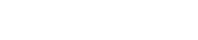高 中1对1课程高三课程

```

```
`	`

```

```
`	`

```

```
`	`

```

```
`	`

```

```
`	`

```

```
`	`

```

```
`	`

```

```
`	`

```

```
`	`

```

```
`	`

```

```
`	`

```

```
`	`

```

```
`	`

```

```
`	`

```

```
`	`

```

```
`	`

```

```
`	`

```

```
`	`

```

```
`	`

```

```
`	`

```

```
`	`

```

```
`	`

```

```
`	`

```

```
`	`

```

```
`	`

```

```
`	`

```

```
`	`

```

```
`	`

```

```
`	`

```

```
`	`

```

```
`	`

```

```
`	`

```

```
`	`

```

```
`	`

```

```
`	`

```

```
`	`

```

```
`	`

```

```
`	`

```

```
`	`

```

```
`	`

```

```
`	`

```

```
`	`

```

```
`	`

```

```
`	`

```

```
`	`

```

```
`	`

```

```
`	`

```

```
`	`

```

```
`	`

```

```
`	`

```

```
`	`

```

```
`	`

```

```
`	`

```

```
`	`

```

```
`	`

```

```
`	`

```

```
`	`

```

```
`	`

```

```
`	`

```

```
`	`

```

```
`	`

```

```
`	`

```

```
`	`

```

```
`	`

```

```
`	`

```

```
`	`

```

```
`	`

```

```
`	`

```

```
`	`

```

```
`	`

```

```
`	`

```

```
`	`

```

```
`	`

```

```
`	`

```

```
`	`

```

```
`	`

```

```
`	`

```

```
`	`

```

```
`	`

```

```
`	`

```

```
`	`

```

```
`	`

```

```
`	`

```

```
`	`

```

```
`	`

```

```
`	`

```

```
`	`

```

```
`	`

```

```
`	`

```

```
`	`

```

```
`	`

```

```
`	`

```

```
`	`

```

```
`	`

```

```
`	`

```

```
`	`

```

```
`	`

```

```
`	`

```

```
`	`

```

```
`	`

```

```
`	`

```

```
`	`

```

```
`	`

```

```
`	`

```

```
`	`

```

```
`	`

```

```
`	`

```

```
`	`

```

```
`	`

```

```
`	`

```

```
`	`

```

```
`	`

```

```
`	`

```

```
`	`

```

```
`	`

```

```
`	`

```

```
`	`

```

```
`	`